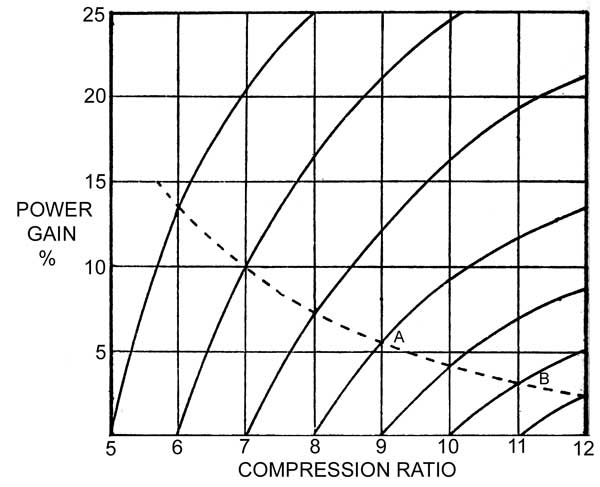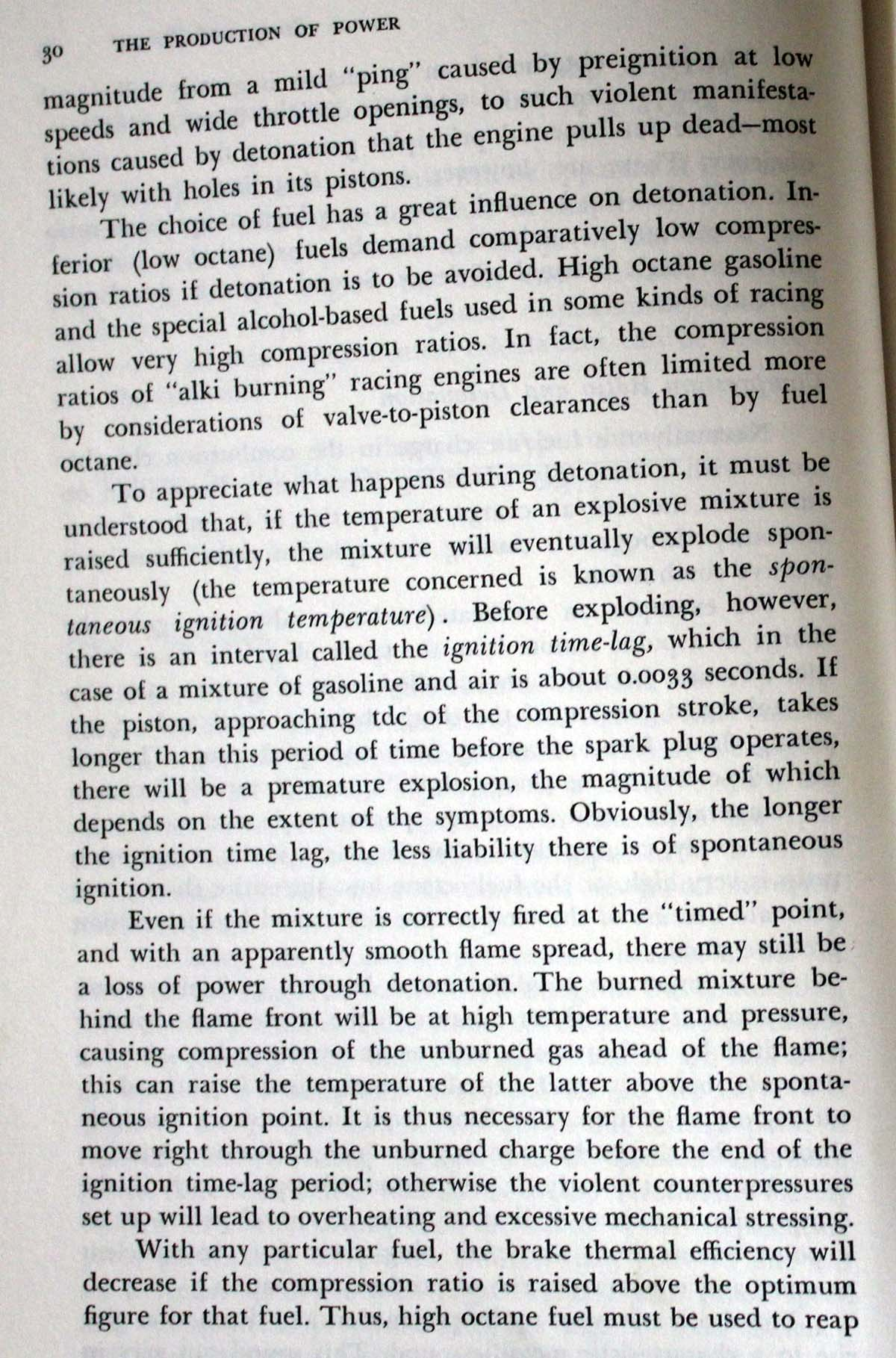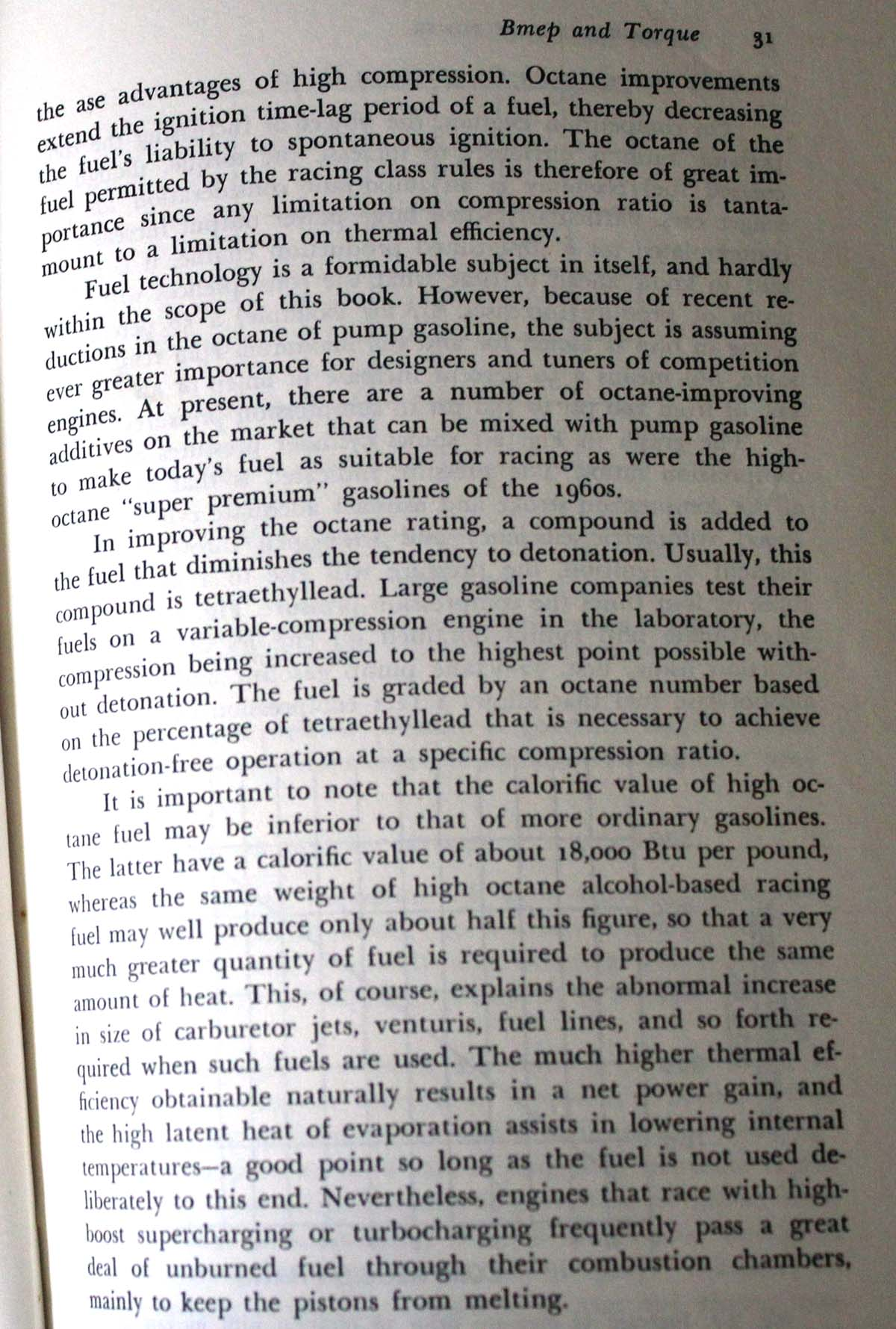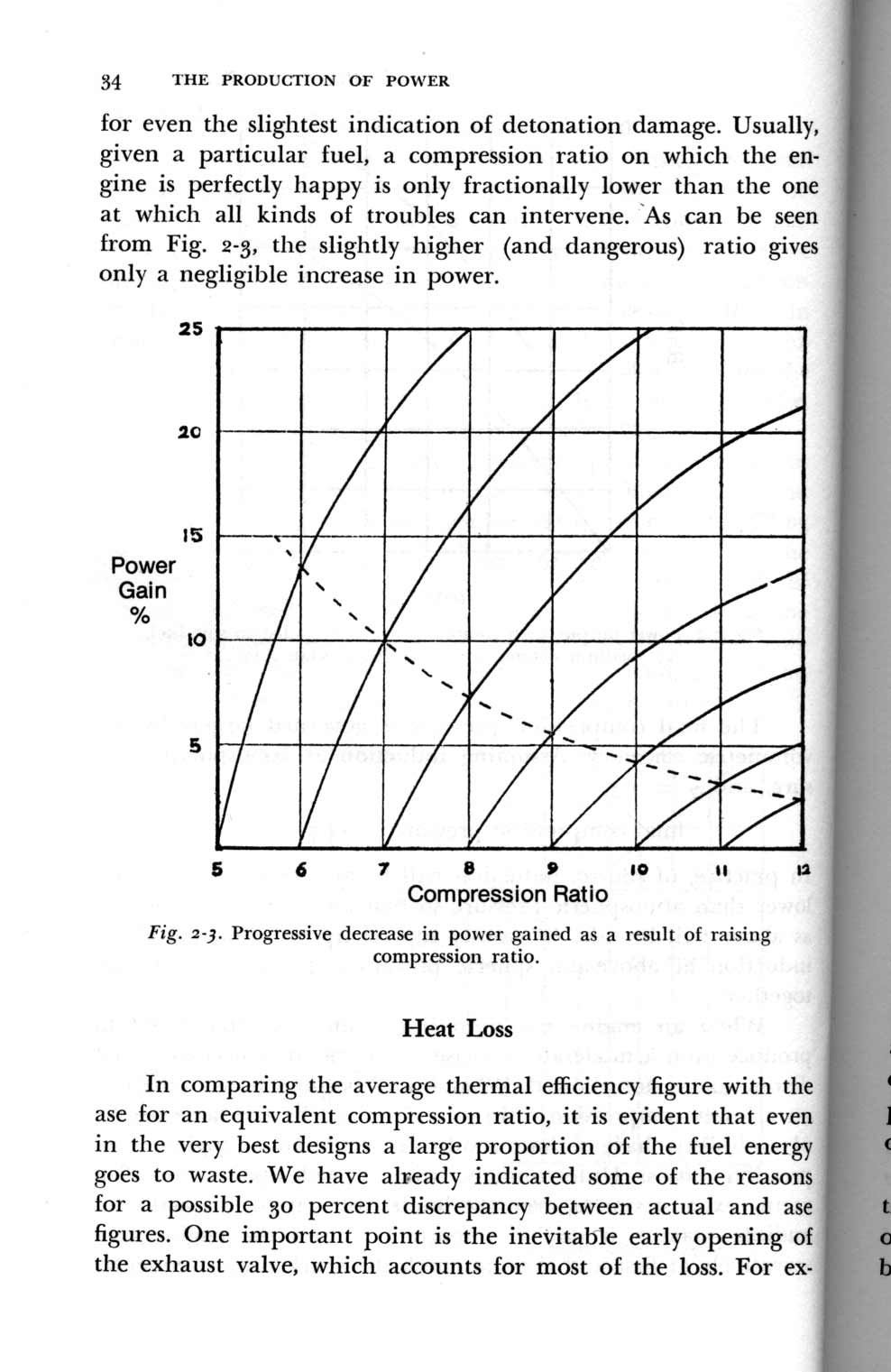# Compression power gain

 Compression power gain [ Home ][ Up ]

 One common question is how much power increases when compression is boosted. The following graph shows the maximum power increase as compression is raised. It assumes fuel octane increases enough to prevent spark knock.The dashed line curve shows the point of next compression number up. Notice how that curve starts curving downward steeply but flattens out as the compression becomes higher and higher. If we look at point A (9:1 compression) as a starting point and go to point B (11:1 compression) the power increases 4% going to 10:1 and 3% going from 10:1 to 11:1. This is a 7% increase total. The formula is